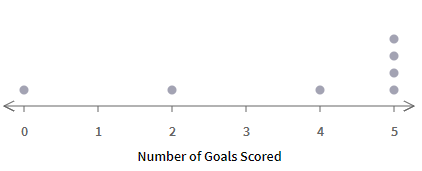ResourcesTestimonials
Plans
ResourcesTestimonials
Plans
Worksheet

# Read And Interpret Dot Plots Worksheet

## 8 problemsIn 6th Grade statistics, students learn dot plots, and one of its essential parts is reading and interpreting the dot plots. Teachers can help them by using the “Read and Interpret Dot Plots Worksheet”. It includes the problems where a dot plot is given and students need to choose the option that represents the data set of the given dot plot or they are asked some questions related to the dot plot. This worksheet is useful for students to develop their understanding of dot plots.

Statistics
6.SP.B.4

For example: Coach Brock is keeping track of the number of goals scored during the team's soccer games.1. How many games were recorded?

2. Which data set represents the points shown in the dot plot?

0, 2, 4, 5

1, 1, 1, 4

0, 2, 4, 5, 5, 5, 5

Solution

1. Count all the dot of dot plot to find the total number of games.

Number of dots = 7

Therefore, 7 games were recorded.

1. In a dot plot, dots above a number represents it occurrence or frequency.

Frequency of 0 = 1

Frequency of 1 = 0

Frequency of 2 = 1

Frequency of 3 = 0

Frequency of 4 = 1

Frequency of 5 = 4

Therefore, the data set for the given dot plot is 0, 2, 4, 5, 5, 5, 5.

For example: Coach Brock is keeping track of the number of goals scored during the team's soccer games.1. How many games were recorded?

2. Which data set represents the points shown in the dot plot?

0, 2, 4, 5

1, 1, 1, 4

0, 2, 4, 5, 5, 5, 5

Solution

Show all

### What teachers are saying about BytelearnWhat teachers are sayingStephen Abate
19-year math teacher
Carmel, CA
Any math teacher that I know would love to have access to ByteLearn.Jennifer Maschino
4-year math teacher
Summerville, SC
“I love that ByteLearn helps reduce a teacher’s workload and engages students through an interactive digital interface.”Rodolpho Loureiro
Dean, math program manager, principal
Miami, FL
“ByteLearn provides instant, customized feedback for students—a game-changer to the educational landscape.”